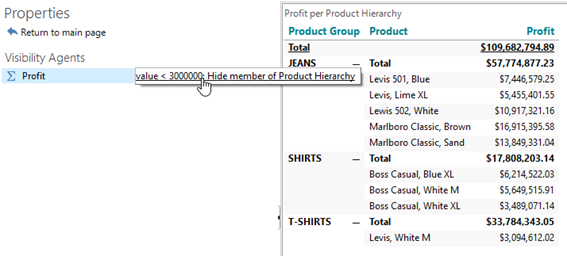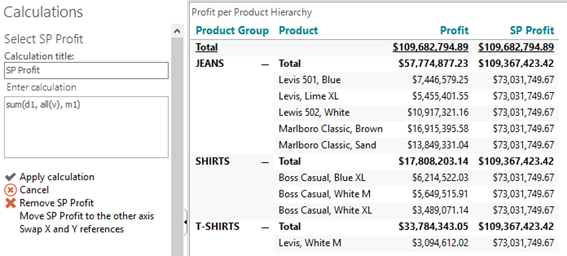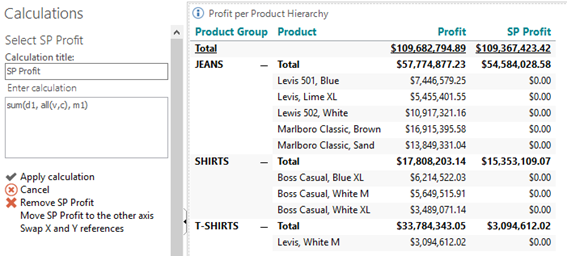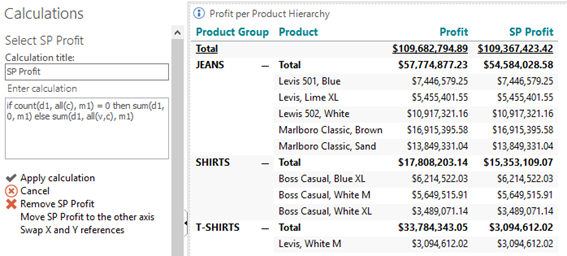# Child Modifier

• Create a new Analysis with a Cross table showing Profit per Product Hierarchy(Product).
• Use a Visibility Agent to hide all products where Profit < 3.000.000. So now we are only seeing the Significant Products (SP).Note: The subtotals are still based on the sums for all products (visible and hidden) for each product group.
• Add a new calculation for the Significant Products, SP Profit = sum(d1, all(v), m1), as a calculation for each product.Note: The result for each product is the total Profit for all visible products (in the first column). The subtotal result for each Product group is the total Profit for all visible Product groups (in the first column).
• Add a Children modifier to the formula, SP Profit = sum(d1, all(v,c), m1).Note: The result for each product is now zero because the products have no children. The subtotal result for each group is now the total Profit for all visible Product group children, i.e. all visible products.
• Finally use an if-then-else statement to produce a satisfying result:

if count(d1, all(c), m1) = 0 then sum(d1, 0, m1) else sum(d1, all(v,c), m1).

Or, in plain text: If the current element has no children then just copy the element's value, otherwise calculate the sum of all its visible children.